# Information Gain From Using Ordinal Instead of Binary Outcomes

RCT
design
ordinal
dichotomization
inference
precision
responder-analysis
sample-size
2018
This article gives examples of information gained by using ordinal over binary response variables. This is done by showing that for the same sample size and power, smaller effects can be detected.
Author
Affiliation

Department of Biostatistics
Vanderbilt University School of Medicine

Published

January 28, 2018

As discussed here, a binary dependent variable Y has minimum statistical information, giving rise to minimal statistical power and precision. This can easily be demonstrated by power or sample size calculations. Consider a pain outcome as an example. Instead of having as an outcome the presence or absence of pain, one can significantly increase power by having several levels of pain severity with the lowest level representing “none”; the more levels the better.

The point about the increase in power can also be made by, instead of varying the effect size, varying the effect that can be detected with a fixed power of 0.9 when the degree of granularity in Y is increased. This is all about breaking ties in Y. The more ties there are, the less statistical information is present. Why is this important in study planning? Here’s an all–too–commmon example. A study is designed to compare the fraction of “clinical responders” between two treatments. The investigator knows that the power of a binary endpoint is limited, and has a fixed budget. So she chooses a more impressive effect size for the power calculation—one that is more than clinically relevant. After the data are in, she finds an apparent clinically relevant improvement due to one of the treatments, but because the study was sized only to detect a super-clinical improvement, the p-value is large and the confidence interval for the effect is wide. Little new knowledge is gained from the study except for how to spend money.

Consider a two-group comparison, with an equal sample size per group. Suppose we want to detect an odds ratio of 0.5 (OR=1.0 means no group effect) for binary Y. Suppose that the probability that Y=1 in the control group is 0.2. The required sample size is computed below.

Code
``````require(Hmisc)
dor    <- 0.5     # OR to detect
tpower <- 0.9     # target power

# Apply OR to p1=0.2 to get p2
p2 <- plogis(qlogis(0.2) + log(dor))
n1 <- round(bsamsize(0.2, p2, power=tpower)['n1'])
n  <- 2 * n1``````

The OR of 0.5 corresponds to an event probability of 0.111 in the second group, and the number of subjects required per group is 347 to achieve a power of 0.9 of detecting OR=0.5.

Let’s now turn to using an ordinal response variable Y for our study. The proportional odds ordinal logistic model is the most widely used ordinal response model. It includes both the Wilcoxon-Mann-Whitney two-sample rank test and binary logistic regression as special cases. If ties in Y could be broken, the proportional odds assumption satisfied, and the sample size per group were fixed at 347, what odds ratio would be detectable with the same power of 0.9?

Before proceeding let’s see how close to 0.9 is the power computed using proportional odds model machinery when Y is binary. The vector of cell probabilities needed by the R `popower` function is the average of the cell probabilities over the two study groups. We write a front-end to `popower` that computes this average given the odds ratio and the cell probabilities for group 1.

Code
``````popow <- function(p, or, n) {
# Compute cell probabilities for group 2 using Hmisc::pomodm
p2 <- pomodm(p=p, odds.ratio=or)
pavg <- (p + p2) / 2
popower(pavg, odds.ratio=or, n=n)
}
z <- popow(c(0.8, 0.2), or=dor, n=2 * n1)
z``````
``````Power: 0.911
Efficiency of design compared with continuous response: 0.394
Approximate standard error of log odds ratio: 0.2098 ``````
Code
``binpopower <- z\$power``

The approximation to the binary case isn’t perfect since the PO model method’s power is a little above 0.9. But it’s not bad.

Let’s write an R function that given everything else computes the OR needed to achieve a given power and configuration of cell probabilities in the control group.

Code
``````g <- function(p, n=2 * n1, power=binpopower) {
f <- function(or) popow(p, or=or, n = n)\$power - power
round(uniroot(f, c(dor - 0.1, 1))\$root, 3)
}
# Check that we can recover the original detectable OR
g(c(0.8, 0.2))``````
`` 0.5``

To break ties in Y we’ll try a number of configurations of the cell probabilities for the control group, and for each configuration compute the OR that can be detected with the same power as computed for the binary Y case using the PO model. We will mainly vary the number of levels of Y. For example, to compute the detectable effect size when the probability that Y=1 of 0.2 is divided into two values of Y with equal probability we use `g(c(0.8, 0.1, 0.1), n)`. Results are shown in the table below.

Code
``````# Function to draw spike histograms of probabilities as html base64 insert
h <- function(p) tobase64image(pngNeedle(p, w=length(p))) ``````
Distinct Y Values Cell Probabilities Detectable OR
2.8 .2 0.5
2.5 .5 0.603
3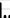.8 .2/2 x 2 0.501
3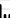.7 .3/2 x 2 0.562
3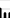.5 .5/2 x 2 0.615
3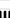1/3 x 3 0.629
4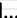.8 .2/3 x 3 0.502
4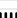1/4 x 4 0.638
5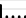0.7 .3/4 x 4 0.563
5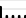0.6 .4/4 x 4 0.597
5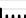0.5 .5/4 x 4 0.618
5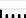0.4 .6/4 x 4 0.631
5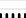1/5 x 5 0.641
6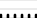1/6 x 6 0.643
7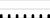1/7 x 7 0.644
10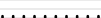1/10 x 10 0.646
694 1/694 x 694 0.647

The last row corresponds to analyzing a continuous variable with the Wilcoxon test with 347 observations per each of the two groups.

When high values of Y (e.g., Y=1 in the binary case) denote an event, and when the control group has a low probability of the event, splitting the high Y-level into multiple ordinal levels does not increase power very much. The real gain in power comes from splitting the more frequent non-event subjects into for example “no event and mild event”. The best power (detectable OR closer to 1.0) comes from having equal probabilities in the cells when averaged over treatment groups, and with at least 5 distinct Y values.

When designing a study, choose a maximum information dependent variable, and attempt to not have more than, say 0.7 of the sample in any one category. But even if the proportion of non-events is large, it does not hurt to break ties among the events. In some cases it will even help, e.g., when the treatment has a larger effect on the more severe events.

The first few lines of `Rmarkdown knitr` markup used to produce the above table are given below.

``````
|Distinct Y Values|                  |Cell Probabilities|Detectable OR     |
|-----------------|------------------|------------------|------------------|
| 2               | `r h(c(.8, .2))` | .8 .2            | `r g(c(.8, .2))` |
| 2               | `r h(c(.5, .5))` | .5 .5            | `r g(c(.5, .5))` |``````